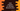# Write a Java program to calculate Discount Price## Java program to calculate discount price :

In this tutorial, we will learn how to calculate the price of a product after discount. We will take both the inputs from user.

### Calculating the price after discount :

To calculate the final price after discount, we will have to multiply the actual price with the discount . e.g. If the actual price is 300 and discount is 10%, final price will be : 300 * 10/100 . 10% means, you will get discount of 10 for a product priced at 100. For a product priced at 1, you will get discount of (10/100). And , for a product priced at 300, you will get discount 300*(10/100).

• Product price 100 = Discount 10
• Product price 1 = Discount 10/100 ( divide by 100 on both side)
• Product price 300 = Discount 300 * (10/100) ( multiply by 300 on both side)

Easy, isn’t it ? Let’s take a look into the program :

### Java Program to calculate Discount :

``````import java.util.Scanner;

public class Main {

/**
* Utility function to print a line
*
* @param line : line to print
*/
static void print(String line) {
System.out.println(line);
}

public static void main(String[] args) {
int price;
int discount;

Scanner sc = new Scanner(System.in);

print("Enter price of the product :");
price = sc.nextInt();

print("Enter Discount of the product :");
discount = sc.nextInt();

int finalPrice = (price * discount) / 100;

print("Final price is " + finalPrice);
}
}``````

### Sample Output :

``````Enter price of the product :
500
Enter Discount of the product :
13
Final price is 65``````

### Using a different method to calculate the Discounted price:

We can also move the final discounted price calculation to a different method like below . This process is helpful to create a utility method and you can call that method from anywhere you want :

``````import java.util.Scanner;

public class Main {

/**
* Utility function to print a line
*
* @param line : line to print
*/
static void print(String line) {
System.out.println(line);
}

static int findFinalPrice(int price, int discount) {
return (price * discount) / 100;
}

public static void main(String[] args) {
int price;
int discount;

Scanner sc = new Scanner(System.in);

print("Enter price of the product :");
price = sc.nextInt();

print("Enter Discount of the product :");
discount = sc.nextInt();

print("Final price is " + findFinalPrice(price, discount));
}
}``````

The output will be same as above Sample Output.